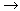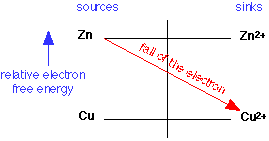The Fall of the electron

Predicting the directions of oxidation-reduction reactions

In oxidation-reduction ("redox") reactions, electrons are transferred from a donor (reducing agent) to an acceptor (oxidizing agent.) But how can you predict whether, or in which direction, such a reaction will actually go? We present here a very simple way of understanding how different redox reactions are related. It is essentially the same as we did for acid-base reactions, in which we saw how protons fall from higher-energy sources (acids) to lower-energy sinks (bases). Similarly, electrons-transfer reactions spontaneously proceed in the direction in which electrons fall (in free energy) from sources (reducing agents) to sinks (oxidizing agents.)

Here is a simple example: will the reaction

Zn + Cu2+Zn2+ + Cu

go in the forward or reverse direction? (Assume equal effective concentrations of the two ions to avoid favoring one or the other direction.) We make use of an electron free energy diagram, the relevant portion of which is shown here:Because electrons have a higher energy on Zn than they do on Cu, copper ions will serve as an electron sink to Zn, and the reaction will go to the right: the Zn gets oxidized to Zn2+, and the Cu2+ is reduced to metallic copper.

After you have digested this example, take a look at the following two pages:

If you already know some electrochemistry, you probably know how to use the Nernst Equation to carry out quantitative calculations. Nevertheless, this is still a very helpful picture when you have to deal with multiple redox systems which occur very commonly in environmental chemistsry, analytical chemistry, and biochemistry.

For further information on this topic, see

Related topic: Fall of the proton - The same idea, showing how to predict the direction of an acid-base reaction and explaining the fundamental distinction between strong and weak acids and bases.